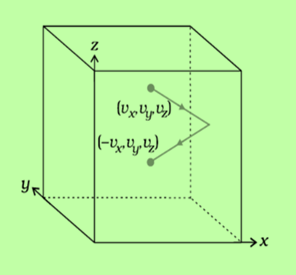# Kinetic Theory of Gases Derivation

## Introduction

This gaseous theory describes the behavior of the gases contained in some vessels. The particles which are molecules or gases are always in random motion as they continuously move and collide with each other and collide with the container also. Also, there is some pressure and temperature within the container.

The path acquired by molecules is straight and the path is known as the mean free path. after the collision, molecules collide with other molecules instantaneously and thus there is always velocity maintained.

## Kinetic Theory of Gases

Gas contains a very huge number of particles whose size is negligible known as molecules. These molecules are in a state of continuous or constant motion or in random motion. During this random motion, molecules continuously move with some velocity and the walls of the container or vessel where it is kept. The collision is so instantaneous.

## Assumption

• Kinetic energy(KE) and momentum(P) will be conserved.

• The size of a gas molecule is negligible(approximately zero).

• No attractive or repulsive force between gas molecules.

• Gas consists of a huge number of tiny particles moving randomly in all possible directions

• center of mass are at rest

• molecules do not exert force on each other or the walls of a container except during a collision.

• The collision between two molecules is perfectly elastic

• molecules of the gases that obey Newton's laws of motion.

## DerivationFig:1 Motion of molecule in a cube

Consider a cubical container containing gas of n number of atoms or molecules peasant in the gas, m mass of each molecule of the gas, and l length of each side of the cube.

Change in the momentum of the molecule,$\mathrm{\Delta P=P_2-P_1}$

$\mathrm{\Delta P=mv_x-(-mv_x)=2mv_x}$

Time for a consecutive strike,

$\mathrm{Time,\:t=\frac{Distance}{Velocity}=\frac{2A}{v_x}}$

Force on the wall,

$\mathrm{Force,\:F=\frac{Change\:in \:momentum}{change\:in\:time}=\frac{\Delta P}{\Delta t}=\frac{2mv_x}{\frac{2A}{v_x}}=\frac{mv_x^{2}}{l}}$

Now,$\mathrm{Pressure,\:P=\frac{force}{area}=\frac{\frac{mv_x^{2}}{l}}{l^2}=\frac{mv_x^{2}}{l^3}}$

$\mathrm{P=\frac{m}{l^2}(v_{x1}^2+v_{x2}^2+v_{x3}^2)}$

$\mathrm{P=\frac{m}{l^2}(Nv_x^{2})}$

$\mathrm{P=\frac{mNv_x^{2}}{v}}$

$\mathrm{{As\:v_x^{2}=v_y^{2}=v_z^{2}}}$ or we write $\mathrm{v^2=3v_x^{2}}$

$\mathrm{v_x^{2}=\frac{1}{3}v^2}$

$\mathrm{PV=\frac{1}{3}mNv^2.......….(1)}$

## The Average Kinetic Energy of the Gas Molecule

As we know from the perfect gas equation

$\mathrm{PV=nRT ........….(2)}$

Equating eq (1) and(2), we get

$\mathrm{\frac{1}{3}mV^2=nRT}$

Multiplying and dividing by 2

$\mathrm{nRT=\frac{1}{2}\frac{2}{3}mv^2}$

$\mathrm{\frac{1}{2}mv^2=\frac{3}{2}\frac{nRT}{N}}$

$\mathrm{\frac{1}{2}mv^2=\frac{3}{2}\frac{RT}{\frac{N}{n}}}$

N - Total no of gas molecules

n - no of moles of gas

$\mathrm{\frac{1}{2}mv^2=\frac{3}{2}\frac{RT}{N_A}\:\:\:\:(N_A=\frac{N}{n})}$

$\mathrm{N_A}$ - Avogadro’s number

R - universal constant

T - temperature

m - mass

v - velocity

Avogadro’s number is the no. of molecules present in one mole of gas

Therefore the average KE of gas is given by

$\mathrm{K.E=\frac{3}{2}kT\:\:\:\:\:(k=\frac{R}{N_A})}$

k - Boltzmann’s constant

For monoatomic molecules, the total internal energy is given by:

$\mathrm{E_{Total}=\frac{3}{2} PV}$

Also, $\mathrm{E_{Total}=\frac{3}{2} NkT}$

$\mathrm{E_{Total}=\frac{3}{2} nRT}$

## Significance of Kinetic Energy

By knowing the Temperature we can directly figure out the mean Kinetic energy of a gas molecule as the temperature is directly proportional to the kinetic energy of the gas molecules.

The molecules or atoms of any gas are considered but yes gas is taken as an ideal gas.

The kinetic theory of gas is very helpful in understanding the macroscopic parameters like pressure, volume, temperature, or microscopic parameters like kinetic energy, momentum, velocity, etc, and vice-versa of the particles.

## Conclusion

The kinetic theory of gas is very helpful in understanding the macroscopic properties or microscopic properties of the particles. By knowing the temperature we can easily find average kinetic energy as kinetic energy is directly proportional to the temperature. All this will be studied under ideal gas.

## FAQs

Q1. The speed of the four balls which are rolled is $\mathrm{1ms^{-1},2ms^{-1},2ms^{-1},\:and \:4ms^{-1}}$ respectively. What will be their root mean square speed?

Ans. As we know, $\mathrm{v_{rms}=\sqrt{\frac{v_1^{2}+v_1^{2}+v_1^{2}+v_1^{2}}{4}}}$

$\mathrm{v_{rms}=\sqrt{\frac{1+4+9+16}{4}}}$

$\mathrm{v_{rms}=2.5m/s(approximately)}$

Q2. Which factors the average kinetic energy depends on?

Ans. Temperature is the factor that only affects the kinetic energy as the temperature is directly proportional to the kinetic energy of the particles contained in a vessel or container.

Q3. is the temperature less than absolute zero possible?

Ans. No, the temperature can’t be less than absolute zero. We can say that so because if the temperature becomes zero then the mean square velocity will also be zero and we know that molecules can’t be negative so it is not possible.

Q4. The container contains 1 mole of gas of temperature T1 and pressure is given as P. What will be the pressure of the identical container containing 1 mole of the same gas at temperature 2T?

Ans. As we know, the ideal gas equation

$\mathrm{PV=nRT}$

$\mathrm{\frac{PV}{T}=nR}$ or constant

So $\mathrm{\frac{P_1V_1}{T_1}=\frac{P_2V_2}{T_2}}$

$\mathrm{P_{2}=\frac{P_1V_1}{T_1}\times \frac{T_2}{v_2}}$

$\mathrm{P_{2}=\frac{PV}{T}\times \frac{2T}{v}}$ (As identical vessel we have, $\mathrm{V_1=V_2=V}$)

$\mathrm{P_{2}=2P}$

Therefore the pressure becomes double as the temperature gets double.

Q5. “Real gases are behaving as an ideal gas at very low pressure and of high temperature”. Explain the statement.

Ans. In an ideal gas, the molecules have zero volume with zero intermolecular forces.

At the low pressure(P), the amount of gas is so much higher than that of the volume of molecules. As a result, the volume of molecules is insignificant as we compare it with the volume of the gases. And at high temperatures(T), the energy of the molecules or atoms gets very high, that is the effect of intermolecular forces can be ignored. Thus at low pressure and high-temperature gases behave as an ideal gas.

Q6. A container with He gas contains 2 moles of temperature 10°C. Calculate the root mean square speed of the atoms. Assume Helium behaves as an ideal gas.

Ans. Given: no. of molecules, n = 2

Temperature, T = 273 + 10 = 283°C

Universal gas value, R = 8.31 J/mol

as we know,

Molecular mass of helium(He) $\mathrm{= 4\: g/mol = 4\times\:10^{-3} \:kg/mol}$ root mean speed,

$\mathrm{v_{rms}=\sqrt{\frac{3RT}{M}}}$

$\mathrm{v_{rms}=\sqrt{\frac{3\times 8.31 \times 283}{4\times 10^{-3}}}}$

$\mathrm{v_{rms}=1.76 \times \:10^6\: m/s}$

Therefore root mean square of helium gas at 10°C is $\mathrm{1.76 \times \:10^{6}\: m/s}$

Updated on: 10-Jan-2023

743 Views